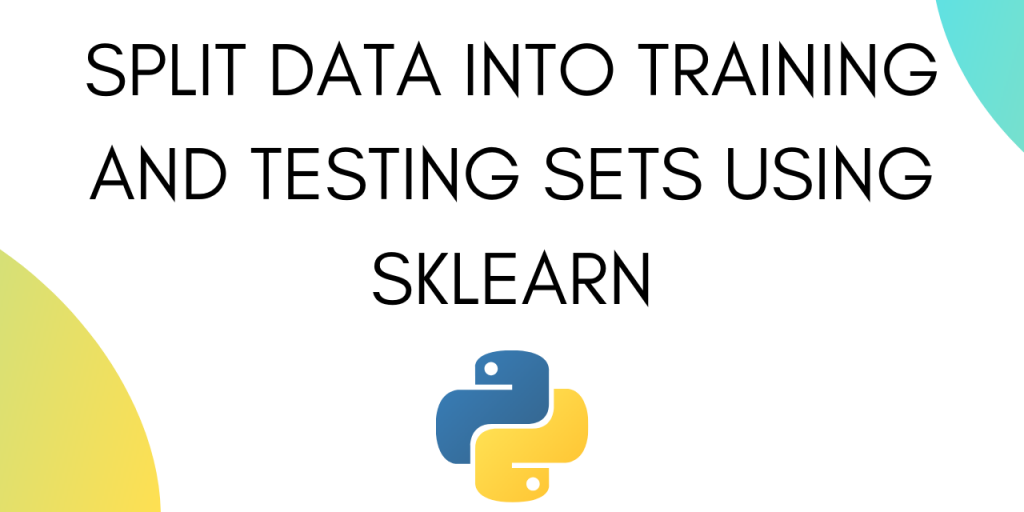# How to Split Data into Training and Testing Sets in Python using sklearn?In machine learning, it is a common practice to split your data into two different sets. These two sets are the training set and the testing set. As the name suggests, the training set is used for training the model and the testing set is used for testing the accuracy of the model.

In this tutorial, we will:

• first, learn the importance of splitting datasets
• then see how to split data into two sets in Python

## Why do we need to split data into training and testing sets?

While training a machine learning model we are trying to find a pattern that best represents all the data points with minimum error. While doing so, two common errors come up. These are overfitting and underfitting.

### Underfitting

Underfitting is when the model is not even able to represent the data points in the training dataset. In the case of under-fitting, you will get a low accuracy even when testing on the training dataset.

Underfitting usually means that your model is too simple to capture the complexities of the dataset.

### Overfitting

Overfitting is the case when your model represents the training dataset a little too accurately. This means that your model fits too closely. In the case of overfitting, your model will not be able to perform well on new unseen data. Overfitting is usually a sign of model being too complex.

Both over-fitting and under-fitting are undesirable.

### Should we test on training data?

Ideally, you should not test on training data. Your model might be overfitting the training set and hence will fail on new data.

Good accuracy in the training dataset can’t guarantee the success of your model on unseen data.

This is why it is recommended to keep training data separate from the testing data.

The basic idea is to use the testing set as unseen data.

After training your data on the training set you should test your model on the testing set.

If your model performs well on the testing set, you can be more confident about your model.

## How to split training and testing data sets in Python?

The most common split ratio is 80:20.

That is 80% of the dataset goes into the training set and 20% of the dataset goes into the testing set.

Before splitting the data, make sure that the dataset is large enough. Train/Test split works well with large datasets.

Let’s get our hands dirty with some code.

### 1. Import the entire dataset

We are using the California Housing dataset for the entirety of the tutorial.

Let’s start with importing the data into a data frame using Pandas.

You can install pandas using the pip command:

```pip install pandas
```

Import the dataset into a pandas Dataframe using :

```import pandas as pd
```

Let’s treat the median_income column as the output (Y).

```y= housing.median_income
```

Simultaneously we will have to drop the column from dataset to form the input vector.

```x=housing.drop('median_income',axis=1)
```

You can use the .head() method in Pandas to see what the input and output look like.

```x.head()
```
```y.head()
```

Now that we have our input and output vectors ready, we can split the data into training and testing sets.

### 2. Split the data using sklearn

To split the data we will be using train_test_split from sklearn.

train_test_split randomly distributes your data into training and testing set according to the ratio provided.

Let’s see how it is done in python.

```x_train,x_test,y_train,y_test=train_test_split(x,y,test_size=0.2)
```

Here we are using the split ratio of 80:20. The 20% testing data set is represented by the 0.2 at the end.

To compare the shape of different testing and training sets, use the following piece of code:

```print("shape of original dataset :", housing.shape)
print("shape of input - training set", x_train.shape)
print("shape of output - training set", y_train.shape)
print("shape of input - testing set", x_test.shape)
print("shape of output - testing set", y_test.shape)
```

This gives the following output.

## The Complete Code

The complete code for this splitting training and testing data is as follows :

```import pandas as pd

#output
y= housing.median_income

#input
x=housing.drop('median_income',axis=1)

#splitting
x_train,x_teinst,y_train,y_test=train_test_split(x,y,test_size=0.2)

#printing shapes of testing and training sets :
print("shape of original dataset :", housing.shape)
print("shape of input - training set", x_train.shape)
print("shape of output - training set", y_train.shape)
print("shape of input - testing set", x_test.shape)
print("shape of output - testing set", y_test.shape)

```

## Conclusion

In this tutorial, we learned about the importance of splitting data into training and testing sets. Furthermore, we imported a dataset into a pandas Dataframe and then used sklearn to split the data into training and testing sets.